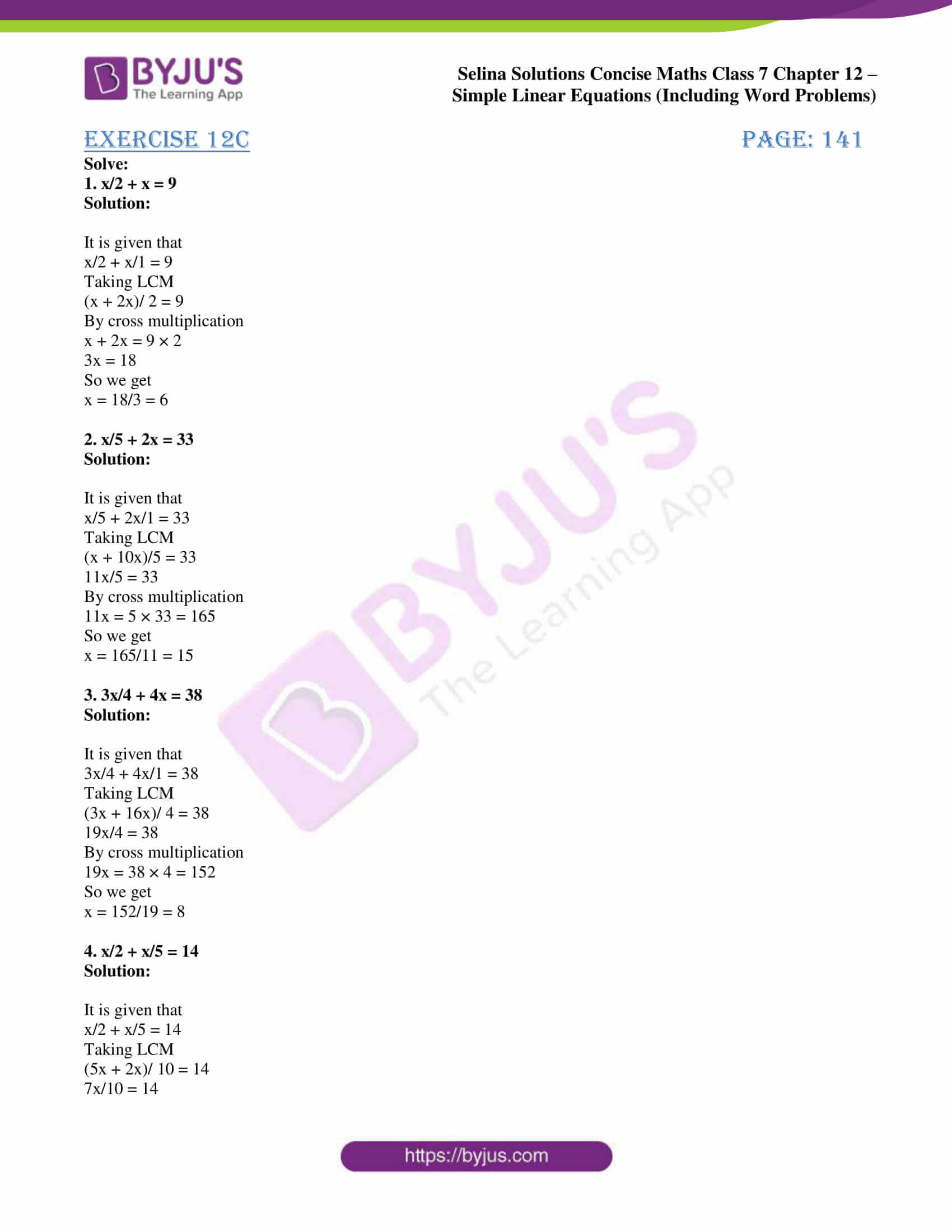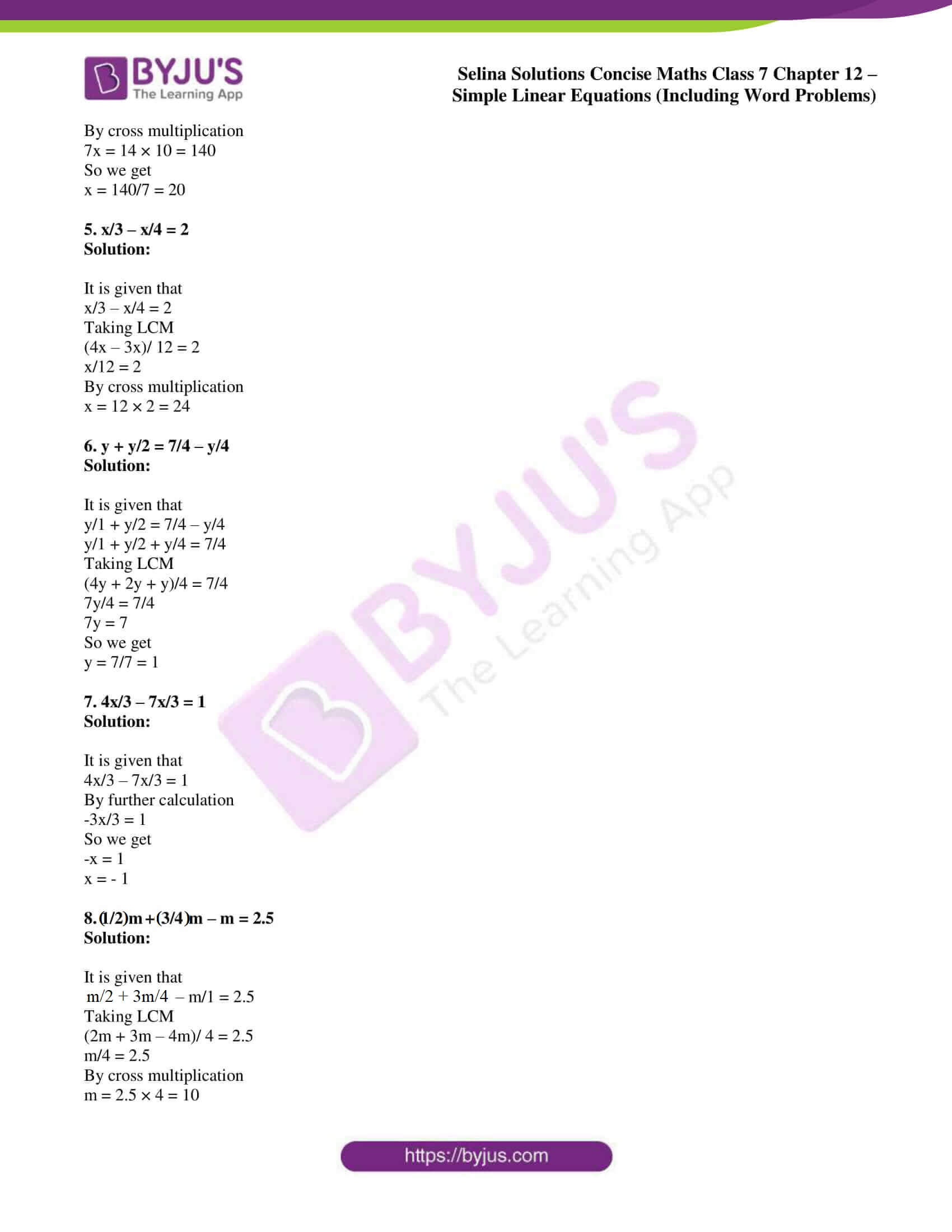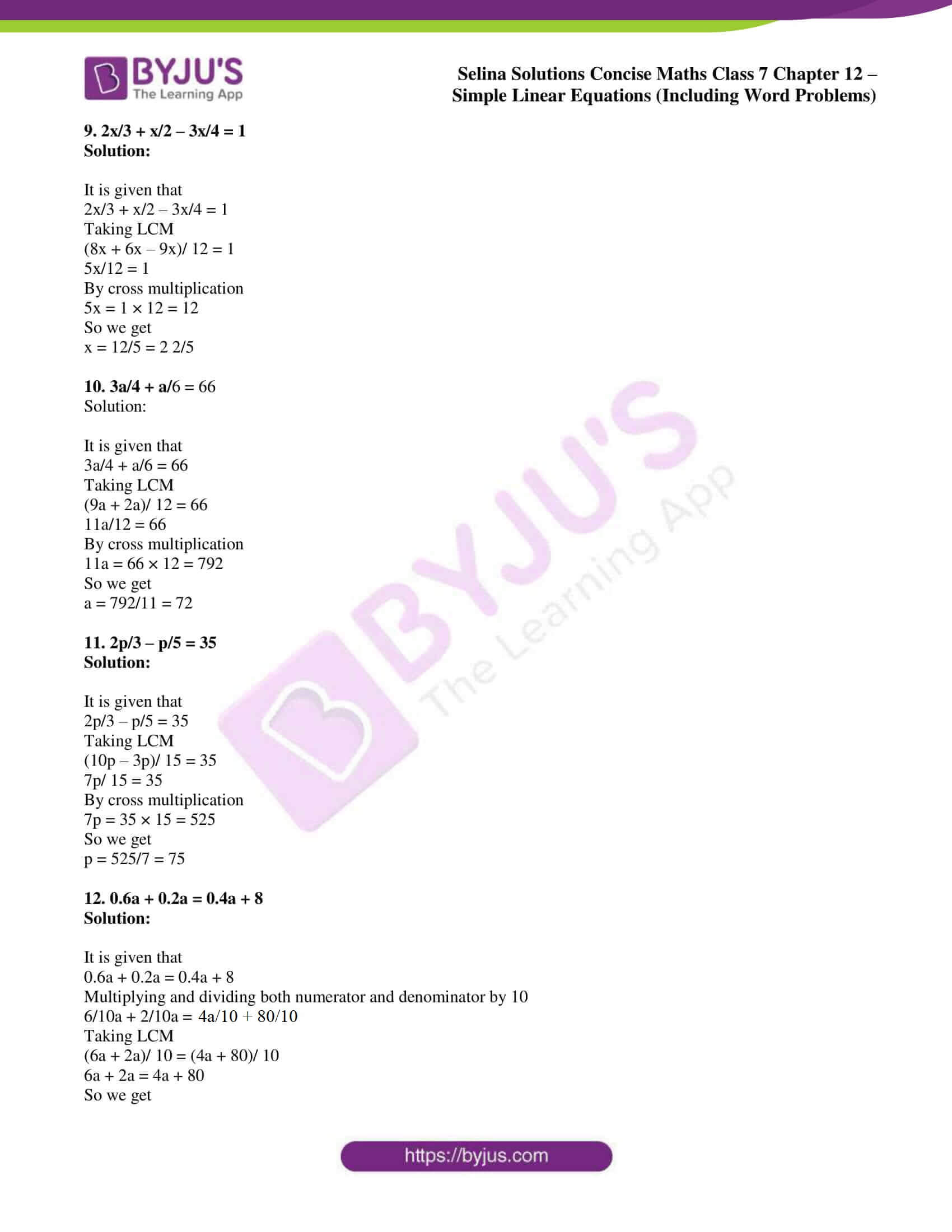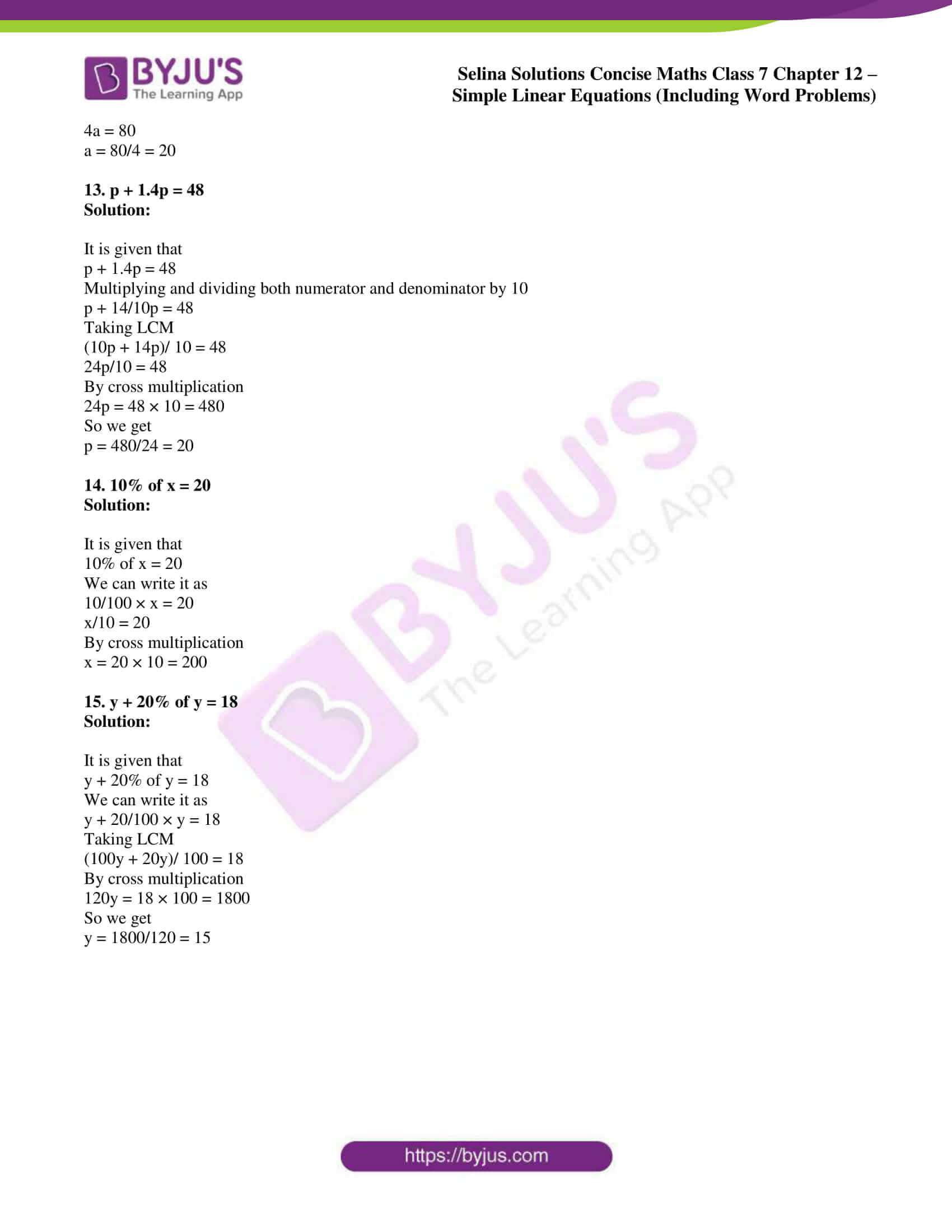# Selina Solutions Concise Maths Class 7 Chapter 12: Simple Linear Equations (Including Word Problems) Exercise 12C

Selina Solutions Concise Maths Class 7 Chapter 12 Simple Linear Equations (Including Word Problems) Exercise 12C is created by highly experienced faculty having vast experience in the education industry. The steps to be followed in solving equations that involve fractions are the main concept discussed under this exercise. Various solved examples are present before the exercise problems to help students analyze the questions that would appear in the annual exam. Students can access Selina Solutions Concise Maths Class 7 Chapter 12 Simple Linear Equations (Including Word Problems) Exercise 12C PDF, from the links which are available below.

## Selina Solutions Concise Maths Class 7 Chapter 12: Simple Linear Equations (Including Word Problems) Exercise 12C Download PDF### Access other exercises of Selina Solutions Concise Maths Class 7 Chapter 12: Simple Linear Equations (Including Word Problems)

Exercise 12A Solutions

Exercise 12B Solutions

Exercise 12D Solutions

### Access Selina Solutions Concise Maths Class 7 Chapter 12: Simple Linear Equations (Including Word Problems) Exercise 12C

#### Exercise 12C page: 141

Solve:

1. x/2 + x = 9

Solution:

It is given that

x/2 + x/1 = 9

Taking LCM

(x + 2x)/ 2 = 9

By cross multiplication

x + 2x = 9 × 2

3x = 18

So we get

x = 18/3 = 6

2. x/5 + 2x = 33

Solution:

It is given that

x/5 + 2x/1 = 33

Taking LCM

(x + 10x)/5 = 33

11x/5 = 33

By cross multiplication

11x = 5 × 33 = 165

So we get

x = 165/11 = 15

3. 3x/4 + 4x = 38

Solution:

It is given that

3x/4 + 4x/1 = 38

Taking LCM

(3x + 16x)/ 4 = 38

19x/4 = 38

By cross multiplication

19x = 38 × 4 = 152

So we get

x = 152/19 = 8

4. x/2 + x/5 = 14

Solution:

It is given that

x/2 + x/5 = 14

Taking LCM

(5x + 2x)/ 10 = 14

7x/10 = 14

By cross multiplication

7x = 14 × 10 = 140

So we get

x = 140/7 = 20

5. x/3 – x/4 = 2

Solution:

It is given that

x/3 – x/4 = 2

Taking LCM

(4x – 3x)/ 12 = 2

x/12 = 2

By cross multiplication

x = 12 × 2 = 24

6. y + y/2 = 7/4 – y/4

Solution:

It is given that

y/1 + y/2 = 7/4 – y/4

y/1 + y/2 + y/4 = 7/4

Taking LCM

(4y + 2y + y)/4 = 7/4

7y/4 = 7/4

7y = 7

So we get

y = 7/7 = 1

7. 4x/3 – 7x/3 = 1

Solution:

It is given that

4x/3 – 7x/3 = 1

By further calculation

-3x/3 = 1

So we get

-x = 1

x = – 1

8. m/2 + 3m/4 – m = 2.5

Solution:

It is given that

m/2 + 3m/4 – m/1 = 2.5

Taking LCM

(2m + 3m – 4m)/ 4 = 2.5

m/4 = 2.5

By cross multiplication

m = 2.5 × 4 = 10

9. 2x/3 + x/2 – 3x/4 = 1

Solution:

It is given that

2x/3 + x/2 – 3x/4 = 1

Taking LCM

(8x + 6x – 9x)/ 12 = 1

5x/12 = 1

By cross multiplication

5x = 1 × 12 = 12

So we get

x = 12/5 = 2 2/5

10. 3a/4 + a/6 = 66

Solution:

It is given that

3a/4 + a/6 = 66

Taking LCM

(9a + 2a)/ 12 = 66

11a/12 = 66

By cross multiplication

11a = 66 × 12 = 792

So we get

a = 792/11 = 72

11. 2p/3 – p/5 = 35

Solution:

It is given that

2p/3 – p/5 = 35

Taking LCM

(10p – 3p)/ 15 = 35

7p/ 15 = 35

By cross multiplication

7p = 35 × 15 = 525

So we get

p = 525/7 = 75

12. 0.6a + 0.2a = 0.4a + 8

Solution:

It is given that

0.6a + 0.2a = 0.4a + 8

Multiplying and dividing both numerator and denominator by 10

6/10a + 2/10a = 4a/10 + 80/10

Taking LCM

(6a + 2a)/ 10 = (4a + 80)/ 10

6a + 2a = 4a + 80

So we get

4a = 80

a = 80/4 = 20

13. p + 1.4p = 48

Solution:

It is given that

p + 1.4p = 48

Multiplying and dividing both numerator and denominator by 10

p + 14/10p = 48

Taking LCM

(10p + 14p)/ 10 = 48

24p/10 = 48

By cross multiplication

24p = 48 × 10 = 480

So we get

p = 480/24 = 20

14. 10% of x = 20

Solution:

It is given that

10% of x = 20

We can write it as

10/100 × x = 20

x/10 = 20

By cross multiplication

x = 20 × 10 = 200

15. y + 20% of y = 18

Solution:

It is given that

y + 20% of y = 18

We can write it as

y + 20/100 × y = 18

Taking LCM

(100y + 20y)/ 100 = 18

By cross multiplication

120y = 18 × 100 = 1800

So we get

y = 1800/120 = 15